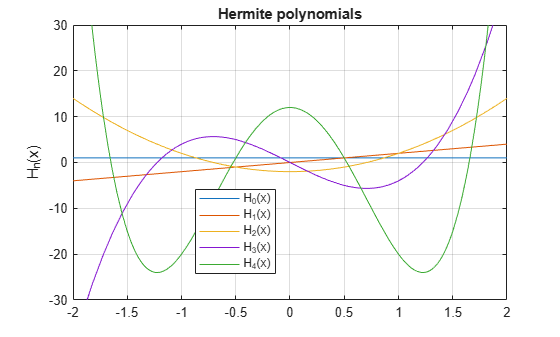# hermiteH

Hermite polynomials

## Syntax

``hermiteH(n,x)``

## Description

example

````hermiteH(n,x)` represents the `n`th-degree Hermite polynomial at the point `x`.```

## Examples

collapse all

Find the first five Hermite polynomials for the variable `x`.

```syms x hermiteH([0 1 2 3 4], x)```
```ans = [ 1, 2*x, 4*x^2 - 2, 8*x^3 - 12*x, 16*x^4 - 48*x^2 + 12]```

Depending on whether the input is numeric or symbolic, `hermiteH` returns numeric or exact symbolic results.

Find the value of the fifth-degree Hermite polynomial at `1/3`. Because the input is numeric, `hermiteH` returns numeric results.

`hermiteH(5,1/3)`
```ans = 34.2058```

Find the same result for exact symbolic input. `hermiteH` returns an exact symbolic result.

`hermiteH(5,sym(1/3))`
```ans = 8312/243```

Plot the first five Hermite polynomials.

```syms x y fplot(hermiteH(0:4,x)) axis([-2 2 -30 30]) grid on ylabel('H_n(x)') legend('H_0(x)', 'H_1(x)', 'H_2(x)', 'H_3(x)', 'H_4(x)', 'Location', 'Best') title('Hermite polynomials')```## Input Arguments

collapse all

Degree of the polynomial, specified as a nonnegative integer, symbolic variable, expression, or function, or as a vector or matrix of numbers, symbolic numbers, variables, expressions, or functions.

Input, specified as a number, vector, matrix, or array, or a symbolic number, variable, array, function, or expression.

collapse all

### Hermite Polynomials

Hermite polynomials are defined by this recursion formula.

`$H\left(0,x\right)=1,\text{ }H\left(1,x\right)=2x,\text{ }H\left(n,x\right)=2xH\left(n-1,x\right)-2\left(n-1\right)H\left(n-2,x\right)$`

Hermite polynomials in MATLAB® satisfy this normalization.

`${\int }_{-\infty }^{\infty }{\left({H}_{n}\left(x\right)\right)}^{2}{\text{e}}^{-{x}^{2}}dx={2}^{n}\sqrt{\pi }n!$`

## Tips

• `hermiteH` returns floating-point results for numeric arguments that are not symbolic objects.

• `hermiteH` acts element-wise on nonscalar inputs.

• At least one input argument must be a scalar or both arguments must be vectors or matrices of the same size. If one input argument is a scalar and the other one is a vector or a matrix, then `hermiteH` expands the scalar into a vector or matrix of the same size as the other argument with all elements equal to that scalar.

 Hochstrasser, U. W. “Orthogonal Polynomials.” Handbook of Mathematical Functions with Formulas, Graphs, and Mathematical Tables. (M. Abramowitz and I. A. Stegun, eds.). New York: Dover, 1972.